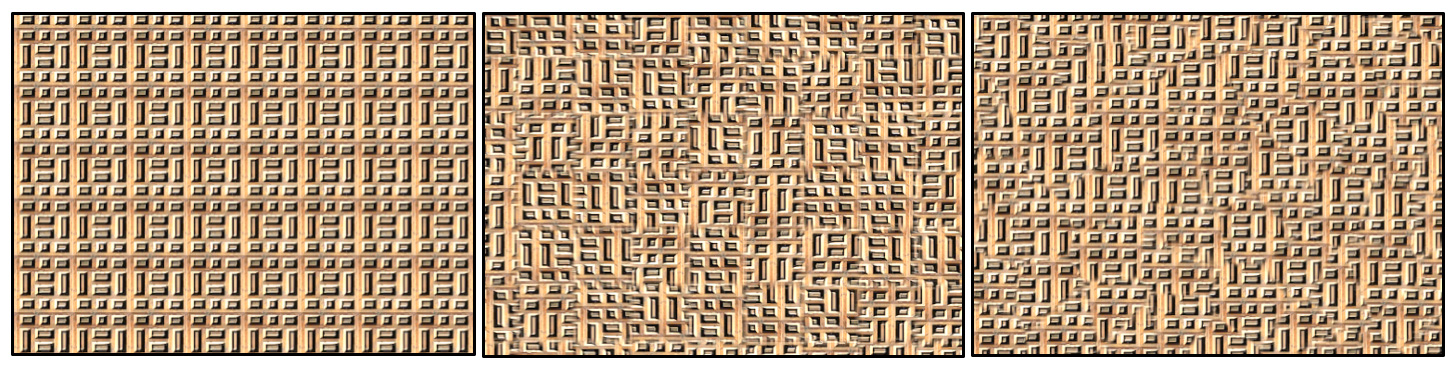# 「Game Tricks」消除纹理重复感的两种方法

Posted by Candycat on November 29, 2016

# 写在前面# 方法一

• 消除每个tile的重复感。这是通过判断当前所处的tile（这可以利用floor(uv)轻松得到），然后给每个tile一个四维的伪随机数，xy表示该tile的翻转方向（即水平和竖直方向上是否要进行mirror），zw表示该tile的平移方向，至此就可以保证每个tile都是不同的了。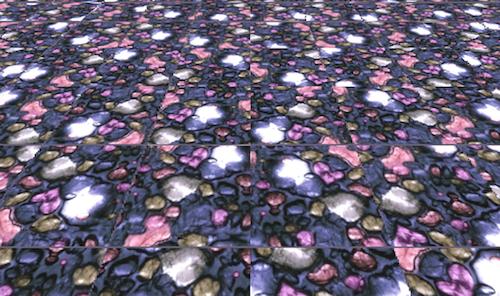• 上一步的结果有两个问题，首先是在tile和tile相交处有明显的接缝问题。这个可以通过算该tile旁边的三个tiles的采样结果，然后靠uv的小数部分判断距离接缝处的距离，并据此来混合四个采样结果，模糊接缝处使得结果看起来比较自然。何时开始混合边界可以当成一个参数来调节。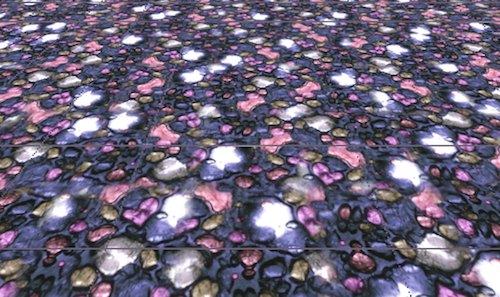• 即便模糊了接缝处，还是会有一些残留的接缝。这些接缝产生的原因是因为我们这种方法会使得uv在tile的边界处产生很大的跳跃，导致mipmaping的时候也会出现跳跃。解决方法就是传递正确的ddx和ddy给tex2D函数，避免uv跳跃即可。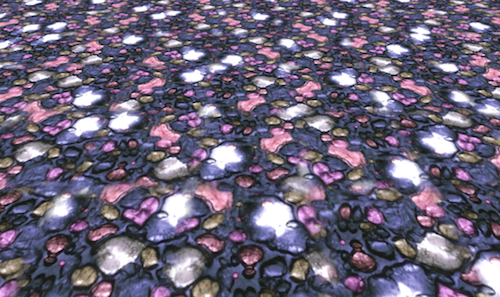1
2
3
4
5
6
7
8
9
10
11
12
13
14
15
16
17
18
19
20
21
22
23
24
25
26
27
28
29
30
31
32
33
34
35
36
37
38
fixed4 texNoTileTech1(sampler2D tex, float2 uv) {
float2 iuv = floor(uv);
float2 fuv = frac(uv);

// Generate per-tile transformation
#if defined (USE_HASH)
float4 ofa = hash4(iuv + float2(0, 0));
float4 ofb = hash4(iuv + float2(1, 0));
float4 ofc = hash4(iuv + float2(0, 1));
float4 ofd = hash4(iuv + float2(1, 1));
#else
float4 ofa = tex2D(_NoiseTex, (iuv + float2(0.5, 0.5))/256.0);
float4 ofb = tex2D(_NoiseTex, (iuv + float2(1.5, 0.5))/256.0);
float4 ofc = tex2D(_NoiseTex, (iuv + float2(0.5, 1.5))/256.0);
float4 ofd = tex2D(_NoiseTex, (iuv + float2(1.5, 1.5))/256.0);
#endif

// Compute the correct derivatives
float2 dx = ddx(uv);
float2 dy = ddy(uv);

// Mirror per-tile uvs
ofa.zw = sign(ofa.zw - 0.5);
ofb.zw = sign(ofb.zw - 0.5);
ofc.zw = sign(ofc.zw - 0.5);
ofd.zw = sign(ofd.zw - 0.5);

float2 uva = uv * ofa.zw + ofa.xy, dxa = dx * ofa.zw, dya = dy * ofa.zw;
float2 uvb = uv * ofb.zw + ofb.xy, dxb = dx * ofb.zw, dyb = dy * ofb.zw;
float2 uvc = uv * ofc.zw + ofc.xy, dxc = dx * ofc.zw, dyc = dy * ofc.zw;
float2 uvd = uv * ofd.zw + ofd.xy, dxd = dx * ofd.zw, dyd = dy * ofd.zw;

// Fetch and blend
float2 b = smoothstep(_BlendRatio, 1.0 - _BlendRatio, fuv);

return lerp(	lerp(tex2D(tex, uva, dxa, dya), tex2D(tex, uvb, dxb, dyb), b.x),
lerp(tex2D(tex, uvc, dxc, dyc), tex2D(tex, uvd, dxd, dyd), b.x), b.y);
}


# 方法二

• 空间划分。整个空间还是会有若干的tile，但是会在每个tile内随机生成一个Voronoi点，每个点对应了一个纹理样式（靠随机平移来区分）。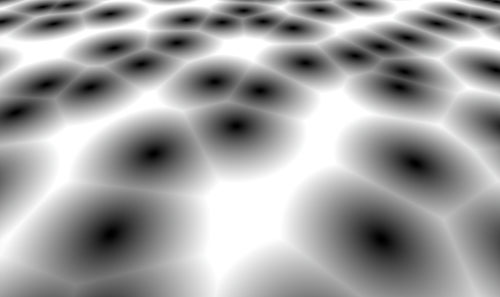• 混合。计算每个像素所在的周围9个Voronoi点，采样得到它们的纹理颜色，混合的时候依靠该像素到每个Voronoi点的距离的高斯衰减值作为混合权重，也就是说，距离Voronoi点越近权重越高。与方法一不同，这种方法其实随时随地都在混合（方法一的混合只发生在边界处），因此采用高斯衰减的好处就在于越靠近高斯衰减权重会迅速升高，使得混合不会造成整体非常模糊。最后，还需要对总体混合权重进行一次归一化，防止颜色失真。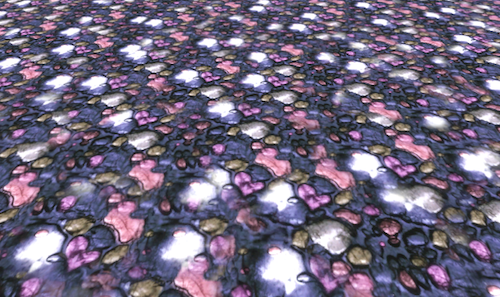1
2
3
4
5
6
7
8
9
10
11
12
13
14
15
16
17
18
19
20
21
22
23
24
25
26
27
28
29
30
31
32
33
fixed4 texNoTileTech2(sampler2D tex, float2 uv) {
float2 iuv = floor(uv);
float2 fuv = frac(uv);

// Compute the correct derivatives for mipmapping
float2 dx = ddx(uv);
float2 dy = ddy(uv);

// Voronoi contribution
float4 va = 0.0;
float wt = 0.0;
float blur = -(_BlendRatio + 0.5) * 30.0;
for (int j = -1; j <= 1; j++) {
for (int i = -1; i <= 1; i++) {
float2 g = float2((float)i, (float)j);
#if defined (USE_HASH)
float4 o = hash4(iuv + g);
#else
float4 o = tex2D(_NoiseTex, (iuv + g + float2(0.5, 0.5))/256.0);
#endif
// Compute the blending weight proportional to a gaussian fallof
float2 r = g - fuv + o.xy;
float d = dot(r, r);
float w = exp(blur * d);
float4 c = tex2D(tex, uv + o.zw, dx, dy);
va += w * c;
wt += w;
}
}

// Normalization
return va/wt;
}


# 写在最后